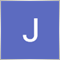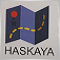# Order Modify problem14

Hi, does anybody know how to open a new trade and then modify all current trades to the same tp, currently my EA can modify the first few trades but after that it just says invalid or unknown ticket.
```int a;
int b;
int total;
double c;
double d;
int cnt;
double firstsellprice;
double secondsellprice;
double thirdsellprice;
int ticket;

void OnTick()
{
total = OrdersTotal();
if (total == 0 && Close >= Open+300*_Point)
{
Open_1st_Sell ();

}
if (total >= 1)
{
get_first_sell();
secondsellprice=(firstsellprice+ 200*_Point);
thirdsellprice =(secondsellprice+ 200*_Point);
Open_2nd_Sell();
}
if (total == 1)
{
Open_2nd_Sell();
Modify_2_sells();
}
if (total == 2)
{
Open_3rd_Sell();
Modify_3_sells();
}
}

// getting sell price
int get_first_sell()
{
for (int i1=0; i1<1; i1++)
{
if (OrderType()==OP_SELL)
{
firstsellprice = OrderOpenPrice();
}
}
return firstsellprice;
}

// sell function
int Open_1st_Sell ()
{
int totalsell = OrdersTotal ();
if (totalsell == 0)
{
OrderSend(Symbol(),OP_SELL,0.1,Bid,3,0,Bid-150*_Point,0,0,0,Green);
}
return 0;
}

// open 2nd sell
int Open_2nd_Sell()
{
int totalsell = OrdersTotal ();
if (totalsell == 1)
{
if (secondsellprice==Bid)
{
OrderSend(Symbol(),OP_SELL,0.15,secondsellprice,3,0,Bid-150*_Point,0,0,0,Green);
}
}
return 0;
}

// open 3rd sell
int Open_3rd_Sell()
{
int totalsell = OrdersTotal ();
if (totalsell == 2)
{
if (thirdsellprice==Bid)
{
OrderSend(Symbol(),OP_SELL,0.38,thirdsellprice,3,0,Bid-150*_Point,0,0,0,Green);
}
}
return 0;
}

// modify 2 sells
int Modify_2_sells()
{
int totalopensells2 = OrdersTotal();
int o2;
int totalsells2 = (OrdersTotal()+OrdersHistoryTotal());
if (totalopensells2 == 2)
{
for (o2; o2<10; o2++)
{
if(OrderType()==OP_SELL)
{
OrderModify(o2,0,0 , secondsellprice-150*_Point, 0, Green);
}
}
}
return 0;
}

// modify 3 sells
int Modify_3_sells()
{
int totalopensells3 = OrdersTotal();
int o3;
int totalsells3 = (OrdersTotal()+OrdersHistoryTotal());
if (totalopensells3 == 3)
{
for (o3; o3<(OrdersHistoryTotal()+OrdersTotal()); o3++)
{
if(OrderType()==OP_SELL)
{
OrderModify(o3,0,0 , thirdsellprice-150*_Point, 0, Green);
}
}
}
return 0;
}
```2215

```// OrderModify(o3,0,0 , thirdsellprice-150*_Point, 0, Green);

OrderModify(OrderTicket(), OrderOpenPrice(),OrderStopLoss(), NormalizeDouble(thirdsellprice-150*_Point,int(MarketInfo(OrderSymbol(),MODE_DIGITS)), 0, CLR_NONE);
```14

Mehmet Bastem:
it works how I wanted it to, thanks so much